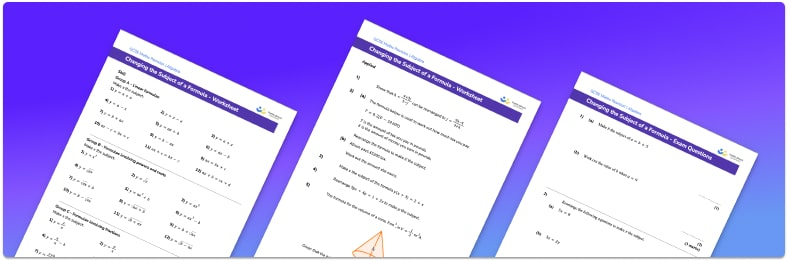# Changing the Subject of a Formula worksheetHelp your students prepare for their Maths GCSE with this free changing the subject of a formula worksheet of 45 questions and answers

• Section 1 of the changing the subject of a formula worksheet contains 36 skills-based changing the subject of a formula questions, in 3 groups to support differentiation
• Section 2 contains 5 applied changing the subject of a formula questions with a mix of worded problems and deeper problem solving questions
• Section 3 contains 4 foundation and higher level GCSE exam questions on changing the subject of a formula
• Answers and a mark scheme for all changing the subject of a formula questions are provided
• Questions follow variation theory with plenty of opportunities for students to work independently at their own level
• All questions created by fully qualified expert secondary maths teachers
• Suitable for GCSE maths revision for AQA, OCR and Edexcel exam boards

• This field is for validation purposes and should be left unchanged.

You can unsubscribe at any time (each email we send will contain an easy way to unsubscribe). To find out more about how we use your data, see our privacy policy.

### Changing the subject of a formula

We use formulae to work out the value of a variable given the values of other variables. For example, the formula Speed = Distance ÷ Time allows us to calculate speed given distance and time. Sometimes we want to work out one of the variables within the formula and to help us do this, we can rearrange the formula to make that variable the subject of the formula. We do this using inverse operations to move variables from one side of the equation to the other. For example, from Speed = Distance ÷ Time, we can multiply by time to get Speed × Time = Distance .

Let’s look at changing the subject of the following formula: a=frac{bx+c}{3}. We could make x the subject. The denominator on the right hand side is 3 so we first need to multiply by 3 to give us 3a=bx+c . Next, subtracting c gives us 3a-c=bx . Finally, dividing by b we get frac{3a-c}{b} = x. Notice now that the right hand side of the formula is just x and the left hand side is the algebraic expression x is equal to. We can swap these and write x=frac{3a-c}{b}.

Looking forward, students can then progress to additional rearranging equations worksheet or more algebra worksheets, for example a sequences worksheet, or a simultaneous equations worksheet.For more teaching and learning support on Algebra our GCSE maths lessons provide step by step support for all GCSE maths concepts.

## Do you have KS4 students who need more focused attention to succeed at GCSE?There will be students in your class who require individual attention to help them succeed in their maths GCSEs. In a class of 30, it’s not always easy to provide.

Help your students feel confident with exam-style questions and the strategies they’ll need to answer them correctly with our dedicated GCSE maths revision programme.

Lessons are selected to provide support where each student needs it most, and specially-trained GCSE maths tutors adapt the pitch and pace of each lesson. This ensures a personalised revision programme that raises grades and boosts confidence.

Find out more